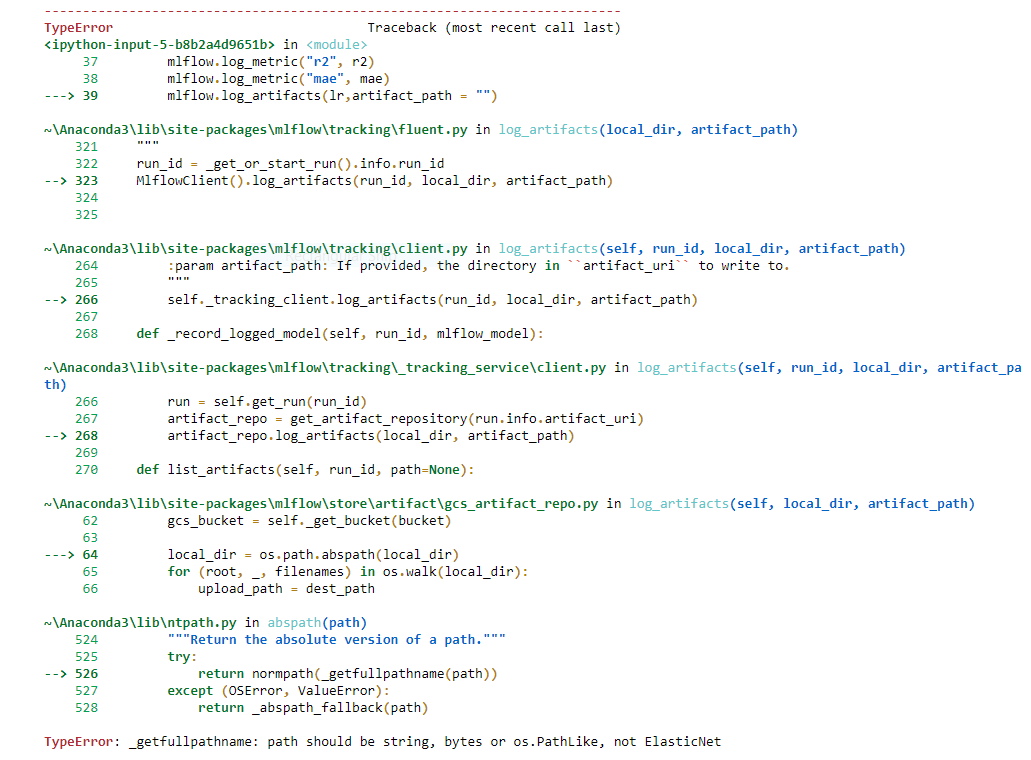# Not able to log artifacts from Windows client to mlflow server running on GCP VM instance

I installed mlflow on GCP VM and started the server by running this command on VM `mlflow server --host x.x.x.x`, here x.x.x.x is the internal IP of the VM

Set the tracking URI using `mlflow.set_tracking_uri("http://x.x.x.x:5000/")`, here x.x.x.x is the external ip of the VM

I'm running this code now to log parameters and artifacts on GCP VM where my mlflow server is running:

``````def eval_metrics(actual, pred):
rmse = np.sqrt(mean_squared_error(actual, pred))
mae = mean_absolute_error(actual, pred)
r2 = r2_score(actual, pred)
return rmse, mae, r2
``````
``````with mlflow.start_run():
lr = ElasticNet(alpha=alpha, l1_ratio=l1_ratio, random_state=42)
lr.fit(train_x, train_y)
predicted_qualities = lr.predict(test_x)
(rmse, mae, r2) = eval_metrics(test_y, predicted_qualities)
print("Elasticnet model (alpha=%f, l1_ratio=%f):" % (alpha, l1_ratio))
print("  RMSE: %s" % rmse)
print("  MAE: %s" % mae)
print("  R2: %s" % r2)
mlflow.log_param("alpha", alpha)
mlflow.log_param("l1_ratio", l1_ratio)
mlflow.log_metric("rmse", rmse)
mlflow.log_metric("r2", r2)
mlflow.log_metric("mae", mae)
mlflow.log_artifacts(lr)
``````

Parameters and Metrics I'm able to get on https://x.x.x.x:5000, where x.x.x.x is external IP of the VM, but at the last line of the code i.e., `mlflow.log_artifacts(lr)` facing the error given below:When executed `mlflow.get_artifact_uri()`, the path returned is `./mlruns/0/6073b44bbac842e5axxxxxxxxxxxxxxxxxx/artifacts`

Is there something wrong with the artifact path and any idea how can I resolve this to log artifacts on VM from code running on local jupyter notebook?